# Triangle calculator

The calculator solves the triangle specified by three of its properties. Each triangle has six main characteristics: three sides a, b, c, and three angles (α, β, γ). The classic trigonometry problem is to specify three of these six characteristics and find the other three. Of course, our calculator solves triangles from any combinations of main and derived properties such as area, perimeter, heights, medians, etc. Usually by the length of three sides (SSS) or side-angle-side or angle-side-angle.

## How does this triangle calculator solve a triangle?

The calculation of the general triangle has two phases:
1. expert phase - which is different for different tasks. From the entered data, the calculator tries to calculate the sizes of three sides of the triangle. He gradually applies the knowledge base to the entered data, which is represented in particular by the relationships between individual parameters of the triangle. These are successively applied and combined, and the parameters of the triangle calculate. Calculator iterate until the triangle has calculated all three sides.
For example, from the given area of the triangle and the corresponding side, the appropriate height is calculated. From the known height and angle, the adjacent side, etc., can be calculated. They use knowledge, e.g., formulas (relations) Pythagorean theorem, Sine theorem, Cosine theorem, Heron's formula, solving equations and systems of equations.
2. The second stage is the calculation of the properties of the triangle from the known lengths of its three sides.

## Examples of how to enter a triangle:

a=3 b=4 c=5 ... triangle calc by three sides a,b,c.
B=45 c=10 a=9 ... triangle calc by two sides a,c and included angle B.
A=25 C=80 b=22
A=35 C=26 a=10
a=3 C=90 c=5 ... how to enter right-angled triangle.
a=3 β=25 γ=45 ... triangle calc if we know the side and two angles.
a=3 β=25 T=12 ... triangle calc, if know side, angle, and area of a triangle.
T=2.5 c=2 b=4 ... find side a if know sides b, c, and area of triangle T.
ma=1 b=2.5 c=2 ... calculation of the triangle if we know one median and any two sides.
ma=1 mb=2.5 mc=2 ... triangle calc by three medians.
ha=220, hb=165 hc=132 ... triangle calc by three heights.
a=7 β=40 mc=5 ... triangle calc by one side, one angle, and one median.
a:b:c=2:3:4 T=2.5 ... a triangle where the known side ratio, and its area.
A:B:C=1:4:5 a=2 ... calculating triangle if we know the ratio of the internal angles and one side.
`What do the symbols mean?a, b, c ... sides BC, AC, ABA, B, C or α, β, γ  ... internal anglesha, hb, hc ... heightsma, mb, mc ... mediansT ... areap ... perimeters ... semiperimeter`

## Triangles in word problems:

• Center traverseDoes the middle traverse indeed bisect the triangle?
• Triangle P2Can a triangle have two right angles?
• AnglesIn the triangle ABC, the ratio of angles is: a:b = 4: 5. The angle c is 36°. How big are the angles a, b?
• Find theFind the third interior angle of the triangle ABC where: α = 48°, γ = 65°.
• Angle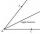Draw angle |∠ ABC| = 130° and built its axis. What angle is between axis angle and arm of angle?
• QuizQ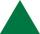An isosceles triangle has two sides of length 7 km and 39 km. How long is a third side?
• Height of right RT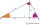The right triangle ABC has a hypotenuse c 9 cm long and a part of the hypotenuse cb = 3 cm. How long is the height of this right triangle?
• MidpointsTriangle whose sides are midpoints of sides of triangle ABC has a perimeter 45. How long is perimeter of triangle ABC?
• Draw a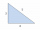Draw a triangle ABC, if you know: alpha = 60° side b = 4 cm side a = 10 cm
• Area of a triangleFind the area of a triangle with a base of 7 mm and a height of 10 mm?
• Similarity of triangles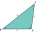If triangle ABC ~ to triangle XYZ, AC = 24, AB = 15, BC = 17, and XY = 9, what is the perimeter of triangle XYZ? Round all sides to 1 decimal place.
• DiagonalCan a rhombus have the same length diagonal and side?
• Centre of massThe vertices of triangle ABC are from the line p distances 3 cm, 4 cm and 8 cm. Calculate distance from the center of gravity of the triangle to line p.
• Triangle ABCConstruct a triangle ABC is is given c = 60mm hc = 40 mm and b = 48 mm analysis procedure steps construction
• Diagonal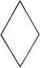Can be a diagonal of diamond twice longer than it side?